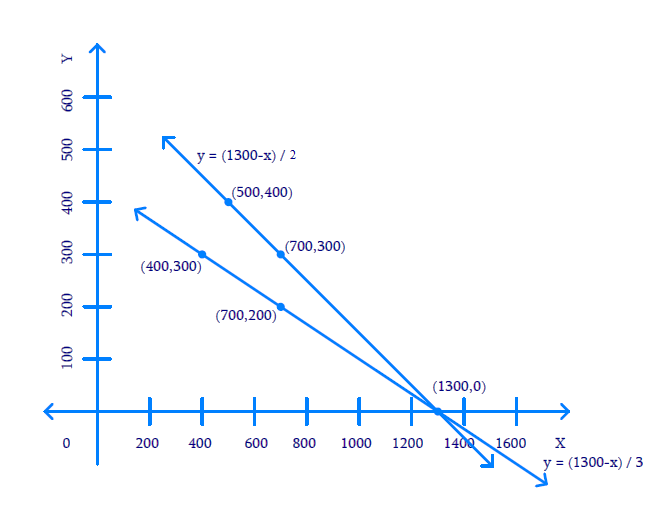# Ex.3.1 Q2 Pair of Linear Equations in Two Variables Solution - NCERT Maths Class 10

Go back to  'Ex.3.1'

## Question

The coach of a cricket team buys $$3$$ bats and 6 balls for $$₹\,3900.$$ Later, she buys another bat and $$3$$ more balls of the same kind for ₹ $$1300.$$ Represent this situation algebraically and geometrically.

Video Solution
Pair Of Linear Equations In Two Variables
Ex 3.1 | Question 2

## Text Solution

What is Known?

(i) Three bats and six balls for ₹ $$3900$$

(ii) One bat and three balls for ₹ $$1300$$

What is Unknown?

Represent the situation geometrically and algebraically

Reasoning:

Assuming the cost of one bat as ₹ $$x$$ and the cost of one ball as ₹ $$y,$$ two linear equations can be formed for the above situation.

Steps:

The cost of $$3$$ bats and 6 balls is ₹ $$3900.$$

Mathematically:

\begin{align}3x + 6y&= 3900\\3(x + 2y)& = 3900\\x + 2y& = 1300\end{align}

Also, the cost of $$1$$ bat and $$3$$ balls is ₹ $$1300.$$

Mathematically:

$x + 3y = 1300$

Algebraic representation where $$x$$ and $$y$$ are cost of bat and ball respectively.

\begin{align}x + 2y &= 1300 \qquad(1)\\x + 3y &= 1300\qquad(2)\end{align}

Therefore, the algebraic representation for equation $$1$$ is:

\begin{align}x + 2y &= 1300\\2y &= 1300-x\\y &= \frac{{1300 - x}}{2}\end{align}

And, the algebraic representation for equation $$2$$ is:

\begin{align}x + 3y &= 1300\\3y &= 1300-x\\y &= \frac{{1300 - x}}{3}\end{align}Let us represent these equations graphically. For this, we need at least two solutions for each equation. We give these solutions in table shown below.

 $$x$$ $$700$$ $$500$$ $$y = \frac{{1300 - x}}{2}$$ $$300$$ $$400$$

 $$x$$ $$400$$ $$700$$ $$y = \frac{{1300 - x}}{3}$$ $$300$$ $$200$$

The graphical representation is as follows.Unit: $$1\,\rm{cm} =$$$$100.$$

The answer is $$(1300, \,0)$$

Learn from the best math teachers and top your exams

• Live one on one classroom and doubt clearing
• Practice worksheets in and after class for conceptual clarity
• Personalized curriculum to keep up with school